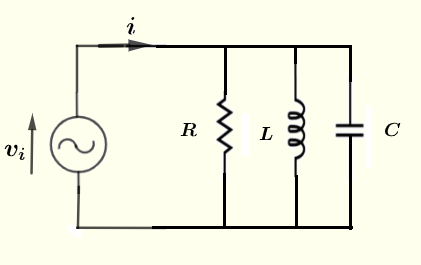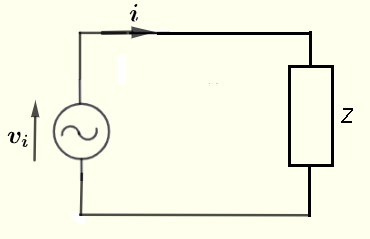# Power Calculator in Parallel RLC

  

A calculator to calculate the average power delivered to a resistor, a capacitor and an inductor in parallel, as shown below, is presented.The calculator gives the impedance of the parallel circuit as a complex numbers in standard form , its modulus and argument, the power factor and the average power.

## Formula for The Average Power Delivered to a pallel RLC CircuitThe general formula of the average power delivered to an impedance $Z$ as shown in the circuit below is given by
$\displaystyle \quad \quad P_a = \dfrac{V_0^2}{2 |Z|} \cos \theta$
where $V_0$ is the peak voltage of the source volatge $v_ i$. $|Z|$ is the modulus of $Z$ and $\theta$ its argument.
The term $\cos \theta$ is called the power factor.
$\omega = 2 \pi f$ , angular frequency in rad/s where $f$ is the frequency of the voltage source.
The formula of the impedance $Z$ of the paralle RLC circuit shown above, in standard complex form, is given by

$\dfrac{1}{Z} = \dfrac{1}{R} + j \omega \; C - j \dfrac{1}{ \omega \; L}$

$|Z| = \dfrac{1} { \sqrt{\dfrac{1}{R^2} + \left(\omega \; C- \dfrac{1}{\omega \; L} \right)^2 }}$

$\theta = - \arctan \left(\dfrac{R(\omega^2 \; L \; C - 1) }{ \omega \; L}\right)$
and in polar form as follows
$Z = |Z| e^{j \theta}$

## Use of the calculator

Enter the resistance, the capacitance, the inductance and the frequency as positive real numbers with the given units then press "calculate".

 Peak Voltage $V_0$ = 5 Volts Resistance R = 1000 mΩ Ω KΩ MΩ Capacitance C = 100 pF nF μF mF F Inductance L = 100 μH mH H Frequency f = 2 GHz MHz kH Hz mHz